# FX Forwards and Futures

## Overview

FX forward contracts are transactions in which counterparties agree to exchange a specified amount of different currencies at some future date, with the exchange rate being set at the time the contract is entered into.  The user is protected from adverse movements in future FX rates, but he also does not benefit from favorable movements.  FX forwards remove uncertainty and are therefore valid instruments for users to mitigate the downside foreign exchange risk for future transactions denominated in a foreign currency.

FX forward rates, FX spot rates, and interest rates are interrelated by the interest rate parity (IRP) principle.  This principle is based on the notion that there should be no arbitrage opportunity between the FX spot market, FX forward market, and the term structure of interest rates in the two countries.

## Formulas & Technical Details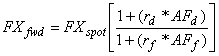where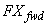= fair forward FX rate (quoted in units of domestic currency per unit of foreign)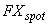= spot FX rate (quoted in units of domestic currency per unit of foreign)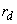= domestic interest rate (for term of forward) quoted on a simple interest basis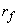= foreign interest rate (for term of forward) quoted on a simple interest basis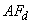= domestic accrual factor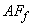= foreign accrual factor

aaFXFwd(FX_spot, rate_dom, rate_for, d_s, d_del, acc_dom, acc_for, stat)

This FINCAD function returns fair basis and fair forward FX rates.

## Examples

### Calculate the 90 day Forward Rate

#### Question

Given the following spot FX spot and money market rates, what should be the theoretical 90 day forward FX rate?

US 90-day Libor = 3.80%aaFXfwd

 Argument Description Example Data Switch FX_spot spot price of underlying currency (domestic per 1 unit of foreign) 1.35 rate_dom domestic rate (for term of forward) 0.045 rate_for foreign rate (for term of forward) 0.038 d_s settlement date 23-Sep-1993 d_del future delivery date 22-Dec-1993 acc_dom accrual method for domestic rate 1 actual / 365 acc_for accrual method for foreign rate 2 actual / 360 stat statistic to be returned 1…2 fair value of forward

Result

 Statistics Description Value 1 Fair FX basis: domestic/foreign 1.3521 2 FX forward price 0.0021

The fair forward FX rate is \$1.3521 CAD/USD.

The fair basis is \$0.0021, i.e., 21 basis points

### Interest Rate Parity

Using the above example to illustrate the principles of IRP, if you borrowed  \$100 CAD at 4.5%, bought USD on the spot market, invested the proceeds in the US at 3.80 %, and sold forward the same amount, you should not be able to extract any arbitrage proceeds from the process.

 1.       Convert the CAD today (100/1.3500) = \$74.074 USD At the end of 90 days: 2.       pay off loan 100 * (1+(0.045*90/365)) =  \$101.11 CAD outflow 3.       USD investment 74.07 * (1+(0.0380*90/360)) = \$74.778 USD inflow

The forward rate should be (101.11/74.778) = 1.3521 CAD/USD

If the forward rate is higher than this value, then you could make riskless profit by following the above strategy.  Suppose the forward rate was 1.3600 CAD/USD.  The profit you would make would be (74.778 * 1.3600) - (101.11) = \$0.588 CAD for every \$100 CAD you borrowed.  By using \$1 million, your riskless profit would be \$5,880.00.  This cannot persist very long in real markets.

If the forward rate was lower than 1.3521, then the reverse strategy can be used.  Suppose the forward rate was 1.3500.  You would then borrow \$100 USD, buy CAD on the spot market, and invest in the Canadian money market, and buy the USD forward to pay off the loan.  This is shown as follows:

 1.       Convert to CAD today 100 * 1.3500 = \$135.00 CAD 90 days from today 2.       pay off loan 100 * (1 + (0.0380 * 90/360)) = 100.95 USD outflow 3.       CAD investment 135 * (1 + (0.0450 * 90/365)) =  136.50 CAD inflow 4.       purchase USD forward 136.50 /1.3500 = 101.11 USD net profit (per \$100 USD) 101.11 - 100.95 = \$0.16 USD

### Quoting Conventions

The previous example was somewhat oversimplified.  There was no bid-ask spread in the spot FX rate and the loan and investment interest rates were assumed equal.  In reality, to calculate valid forward prices one needs to have the valid bid-ask prices of the spot rates, and separate loan and investment interest rates.

Forward rates can be quoted in two ways, as an "outright" quote, or as forward points  (also called a swap rate).  The outright quote is simply a bid-ask price same as the spot market quotes.  The forward points are the amount that needs to be added to or subtracted from the spot rates.  The following illustrates the two quoting methods:

 spot rates 1.3500 - 1.3505 CAD/USD forward points 90 - 95 outright forward rates 1.3590 - 1.3600 CAD/USD

FX forward points can be calculated using the function aaFXfwd() with the statistic switch set to 1 (fair basis).

As previously discussed, the forward rates are closely related to the spot rates and interest rates of the two countries.  A result of the IRP theory is that for the country with the higher interest rate, its currency is weaker in the forward market than in the spot market.  As shown in the previous example, the Canadian interest rate was higher than the US interest rate, and the resulting theoretical forward rate was 1.3521 CAD/USD, compared with the spot rate of 1.3500 CAD/USD.  The terms premium and discount refer to whether the forward rates are higher or lower than the spot rates.  A premium means the forward price is higher than the spot price and a discount means lower.  In this case the forward rate is then at a premium of 21 points.

 Example of a premium Spread spot rates 1.3500 - 1.3505 CAD/USD 5 points forward points (+90) - (+95) 5 points outright forward rates bid = 1.3500 + 0.0090 = 1.3590 ask = 1.3505 + 0.0095 = 1.3600 1.3590 - 1.3600 CAD/USD 10 points

#### Example of a discount

spot rates

5 points

forward points

(-90) -  (-95)

5 points

outright forward rates

bid = 0.7405 - 0.0095 = 0.7310

ask = 0.7410 - 0.0090 = 0.7320

10 points

### Detailed Forward Rate Calculation

We will now provide sample calculations of both a premium and a discount forward price using more realistic data.  Consider the following scenario:

 spot rate CAD/USD 1.3500 - 1.3506 spot rate USD/CAD 0.7404 - 0.7407 90 day Canadian interest rates 5.98 % investment 6.02 % loan 90 day US interest rates 3.92 % investment 3.98 % loan

#### Example of a forward premium calculation

If a Canadian company needs to have USD in 90 days, it can buy USD forward, or pursue the following strategy.  Borrow CAD, convert to USD, invest in USD for 90 days.

 Canadian borrowing costs 1 + (0.0602 * (90/365)) = 1.01484 CAD Converting CAD to USD 1.3506 CAD Total cost 1.01484 * 1.3506 = 1.3706 CAD US investment income 1 + (0.0392 * (90/360)) = 1.0098 USD

The forward price required for the two strategies to break-even (i.e. no arbitrage) would be 1.3706 / 1.0098 = 1.3575 CAD/USD.  Note this is the forward ask price of the USD.  The inverse of this (1 / 1.3575 = 0.7366) is then the forward bid price of the CAD (i.e. sell USD / buy CAD).

USD/CAD forward points = 0.7366 - 0.7404 = -0.0038 (a discount)

#### Example of a forward discount calculation

If a US company needs to have CAD in 90 days, it can buy CAD forward, or pursue the following strategy.  Borrow USD, convert to CAD, invest in CAD for 90 days.

 US borrowing costs 1 + (0.0398 * (90/360)) = 1.00995 USD Converting USD to CAD 0.7407 USD Total cost 1.00995 * 0.7407 = 0.7481 USD Canadian investment income 1 + (0.0598 * (90/365)) = 1.01475 CAD

The forward price required for the two strategies to break-even (i.e. no arbitrage) would be 0.7481 / 1.01475 = 0.7372 USD/CAD.  Note this is the forward ask price of the CAD.  The inverse of this (1 / 0.7372 = 1.3565) is then the forward bid price of the USD (i.e. sell CAD / buy USD).

USD/CAD Forward points = 0.7372 - 0.7407 = -0.0035  (a discount)

 To summarize the results: Spread Forward rate CAD/USD 1.3565 - 75 0.0010 Forward points CAD/USD (+0.0065) - (+0.0069) 0.0004 Forward rate USD/CAD 0.7366 - 72 0.0006 Forward points USD/CAD (-0.0035) - (-0.0038) 0.0003

 Recall the spot rates: Spread Spot rate CAD/USD 1.3500 - 1.3506 0.0006 Spot rate USD/CAD 0.7404 - 0.7407 0.0003

## Related Functions

Calculates the domestic repo rate for a FX forward contract

Calculates the foreign repo rate for a FX forward contract

Simulates the FX forward rate and basis for a FX forward contract

Calculates the fair forward FX and fair basis given zero coupon discount factor curves for the domestic and foreign interest rates

Disclaimer

With respect to this document, FinancialCAD Corporation (“FINCAD”) makes no warranty either express or implied, including, but not limited to, any implied warranty of merchantability or fitness for a particular purpose. In no event shall FINCAD be liable to anyone for special, collateral, incidental, or consequential damages in connection with or arising out of the use of this document or the information contained in it. This document should not be relied on as a substitute for your own independent research or the advice of your professional financial, accounting or other advisors.

This information is subject to change without notice. FINCAD assumes no responsibility for any errors in this document or their consequences and reserves the right to make changes to this document without notice.